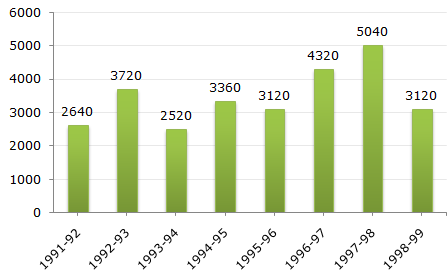# Data Interpretation - Bar Charts

Directions to Solve

The bar graph given below shows the foreign exchange reserves of a country (in million US \$) from 1991 - 1992 to 1998 - 1999.

Foreign Exchange Reserves Of a Country. (in million US \$)1.
The ratio of the number of years, in which the foreign exchange reserves are above the average reserves, to those in which the reserves are below the average reserves is?
2:6
3:4
3:5
4:4
Explanation:

Average foreign exchange reserves over the given period = 3480 million US \$.

The country had reserves above 3480 million US \$ during the years 1992-93, 1996-97 and 1997-98, i.e., for 3 years and below 3480 million US \$ during the years 1991-92, 1993-94, 1994-95, 1995-56 and 1998-99 i.e., for 5 years.

Hence, required ratio = 3 : 5.

2.
The foreign exchange reserves in 1997-98 was how many times that in 1994-95?
0.7
1.2
1.4
1.5
Explanation:

 Required ratio = 5040 = 1.5. 3360

3.
For which year, the percent increase of foreign exchange reserves over the previous year, is the highest?
1992-93
1993-94
1994-95
1996-97
Explanation:

There is an increase in foreign exchange reserves during the years 1992 - 1993, 1994 - 1995, 1996 - 1997, 1997 - 1998 as compared to previous year (as shown by bar-graph).

The percentage increase in reserves during these years compared to previous year are:

 For 1992 - 1993 =(3720 - 2640) x 100% = 40.91%. 2640

 For 1994 - 1995 =(3360 - 2520) x 100% = 33.33%. 2520

 For 1996 - 1997 =(4320 - 3120) x 100% = 38.46%. 3120

 For 1997 - 1998 =(5040 - 4320) x 100% = 16.67%. 4320

Clearly, the percentage increase over previous year is highest for 1992 - 1993.

4.
The foreign exchange reserves in 1996-97 were approximately what percent of the average foreign exchange reserves over the period under review?
95%
110%
115%
125%
Explanation:

Average foreign exchange reserves over the given period

 =1 x (2640 + 3720 + 2520 + 3360 + 3120 + 4320 + 5040 + 3120)million US \$ 8

= 3480 million US \$.

Foreign exchange reserves in 1996 - 1997 = 4320 million US \$.Required percentage =4320 x 100% = 124.14%125%. 3480

5.
What was the percentage increase in the foreign exchange reserves in 1997-98 over 1993-94?
100
150
200
620Increase = (5040 - 2520) = 2520 US \$.Percentage Increase =2520 x 100% = 100%. 2520